# Parameterizing Models of Propositional Calculus Formulas

John McCarthy

Computer Science Department

December 24, 2003

Stanford University

### Abstract:

It is often inadequate that a theory be consistent, i.e. have models. It should have enough models. We discuss parameterizing the set of models in the special case of propositional satisfiability.

A propositional formula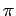in variables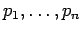is called satisfiable if it has a model, i.e. a tuple of truth values forthat makestrue. Many programs exist for deciding this.

However, we can ask for more than just whether a formula has models; we can ask about its set of models. One way to get a grip on the set of a formula's models is to parametrize it, i.e. to express the variablesof the formulaas propositional expressions in other variables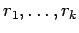, such that arbitrary values ofgive rise to exactly the values ofsatisfying.

Here are some examples.

•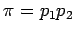. this is a trivial case and the formulas are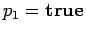and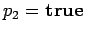.

•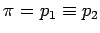. We have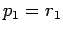and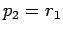.

•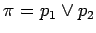. We haveand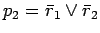.

•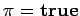. We haveand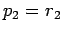.

•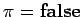. No model.

The number of parameters required depends on the number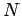of models of. Indeed it is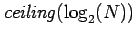, the least it could possibly be. Here's how to construct a parametrization.

Letbe written in disjunctive normal form. Choose enough variables, so that each conjunction is assigned one or more of theconjunctions of the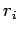and. The assignment can be arbitrary provided each conjunction of the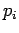s gets a unique conjunction of the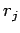s. For each, we write a conditional expression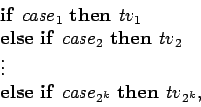(1)

where the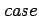s are the r-conjunctions and the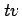's are the truth values thatassumes in that case. The conditional expressions can be converted to propositional expressions if desired, since all the consequents are truth values.

Example:

[We'll use juxtaposition for conjunction to keep the formulas compact.]

Let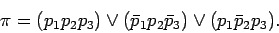(2)

We need only 2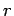variables. These give 4 cases. We can assign the cases to the three terms of (2) arbitrarily, so let's assign tt and tf to the first conjunction of (2) and ft and ff to the two remaining conjunctions. We then have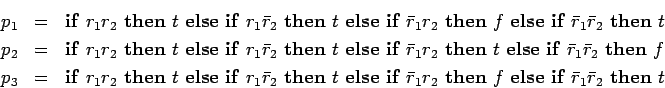Remarks:

1. Presumably the above systematic procedure usually doesn't give the optimal parametrization in terms of length of formula, although it is optimal in the number of r-variables.

2. The parametrization is easy, because we have assumed that the theory is represented by an expressionin disjunctive normal form. If putting the theory in disjunctive normal form leads to an excessively long expression, the parametrization may be more difficult.

3. There is no apparent way of turning a parameterization of the models ofinto a parameterization of the models of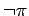.

4. Parameterizing models of modal formulas may offer difficulties, because there will often be an infinity of models of even rather simple formulas. This will depend on the modal logic.

5. Extending the idea to predicate calculus is likely to be reasonable only under some restrictions. Thus parameterizing the models of the axioms for a group is the problem of group classification with its hundred year history. However, Abelian groups are nicely parameterized.

6. Perhaps monadic predicate calculus will be a good domain.

7. Parameterizing the models of nonmonotonic theories may present interesting problems even in the propositional case. 2001 August.

8. There may be straightforward ways of going from a parameterization of a theory to parameterizations of some kinds of elaborations of the theory. This may help with the problem of establishing consistency of the elaborated theory. Thus the mere fact of consistency of a theory may not help in establishing the consistency of an elaborated theory, but a parameterization of the theory may lead to consistency of the elaborated theory via its parameterization. - 2001 August.